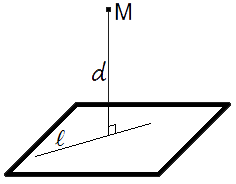Study of mathematics online.
Study math with us and make sure that "Mathematics is easy!"

# Online calculator. Distance from point to plane

This online calculator will help you to find distance between point and plane.

Using this online calculator, you will receive a detailed step-by-step solution to your problem, which will help you understand the algorithm how to find distance between point and plane.

## Distance from point to plane calculatorPlane equation:

x + y + z + = 0

Point coordinates:

M: (
,,
)

You can input only integer numbers, decimals or fractions in this online calculator (-2.4, 5/7, ...). More in-depth information read at these rules.